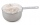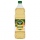# Mixed fractions calculator

The calculator performs basic and advanced operations with mixed numbers, fractions, integers, decimals. Mixed fractions are also called mixed numbers. A mixed fraction is a whole number and a proper fraction combined, i.e. one and three-quarters. The calculator evaluates the expression or solves the equation with step-by-step calculation progress information. Solve problems with two or more mixed numbers fractions in one expression.

## Result:

### 1.5 = 3/2 = 1 1/2

Spelled result in words is one and one half (or three halfs).

### Calculation steps

1. Conversion a decimal number to a fraction: 1.5 = 15/10 = 3/2

a) Write down the decimal 1.5 divided by 1: 1.5 = 1.5/1
b) Multiply both top and bottom by 10 for every number after the decimal point. (For example, if there are two numbers after the decimal point, then use 100, if there are three then use 1000, etc.)
1.5/1 = 15/10
Note: 15/10 is called a decimal fraction.

c) Simplify and reduce the fraction
15/10 = 3 * 5/2 * 5 = 3 * 5/2 * 5 = 3/2

#### Examples:

sum of two mixed numbers: 1 3/4 + 2 3/8
addition of three mixed numbers: 1 3/8 + 6 11/13 + 5 7/8
addition of two mixed numbers: 2 1/2 + 4 2/3
subtracting two mixed numbers: 7 1/2 - 5 3/4
multiplication of mixed numbers: 3 3/4 * 2 2/5
comparing mixed numbers: 3 1/4 2 1/3
changing improper fraction to mixed number: 9/4
What is 3/4 as a mixed number: 3/4
subtracting mixed number and fraction: 1 3/5 - 5/6
sum mixed number and an improper fraction: 1 3/5 + 11/5

## Mixed fractions in word problems:

• Which 5Which mixed number is equivalent to 2.68? A:2 and 6 eighths B:2 and 68 tenths C:2 and 6 over 68
• Mixed2improperWrite the mixed number as an improper fraction. 166 2/3
• Mixed to improperChange the given mixed numbers to improper fraction: five-and-four-over-nine (5 4/9)
• SubtractSubtract two mixed numbers: 3 1/2 -1 3/4Add this two mixed numbers: 1 5/6 + 2 2/11=
• A more than bWhat is 5 1/7 more than 2 3/7?
• Zailene 2Zailene has 8 2/3 cup of flour. She used 5 1/2 cups of flour. How many cups of flour was left to her?
• A scienceA science experiment calls for making 3 and 2/3 cups of distilled water with 1 and 3/5 cups of vinegar and 2/3 cups of liquid detergent. How much liquid in all and cups is needed?
• Perimeter - rectangleFind the perimeter of the rectangle. A rectangle with a base of (3 5/6) m and a height of (2 3/7) m Its perimeter is x= meters. (Use fraction in your answer. )Add this two mixed numbers: 1 5/6 + 2 2/11=Add two mixed fractions: 2 4/6 + 1 3/62 and 1/8th plus 1 and 1/3rd =A number, X, is subtracted from 8 1/4. The result is 12 3/5. What is the value of X?Tyron attended his online class 2 hours a day. He already attend 1 3/4 hours, how many more hours will Tyron attend his class?2 3/5 of 1430+? = 1900. How to do this problemThe product of the two numbers is 15 ⁵/₆. If one of the numbers is 5 ²/₃, find the other.The sum of two mixed numbers is 15½. One of the number is 5 ¾, what is the other?